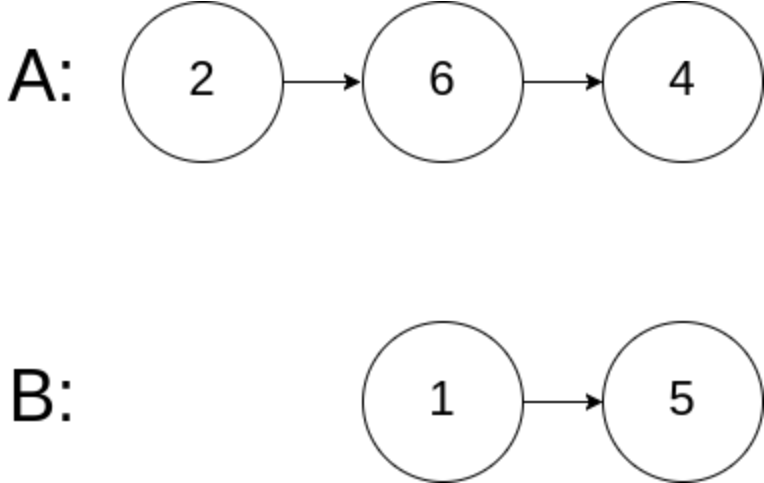# 160. 相交链表

``````输入：intersectVal = 8, listA = [4,1,8,4,5], listB = [5,0,1,8,4,5], skipA = 2, skipB = 3

``````

``````输入：intersectVal = 2, listA = [0,9,1,2,4], listB = [3,2,4], skipA = 3, skipB = 1

````````````输入：intersectVal = 0, listA = [2,6,4], listB = [1,5], skipA = 3, skipB = 2

``````

• listA 中节点数目为 m
• listB 中节点数目为 n
• 0 <= m, n <= 3 * 10^4
• 1 <= Node.val <= 10^5
• 0 <= skipA <= m
• 0 <= skipB <= n
• 如果 listA 和 listB 没有交点，intersectVal 为 0
• 如果 listA 和 listB 有交点，intersectVal == listA[skipA + 1] == listB[skipB + 1]

## 一解

``````# Definition for singly-linked list.
# class ListNode:
#     def __init__(self, x):
#         self.val = x
#         self.next = None

class Solution: